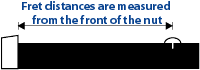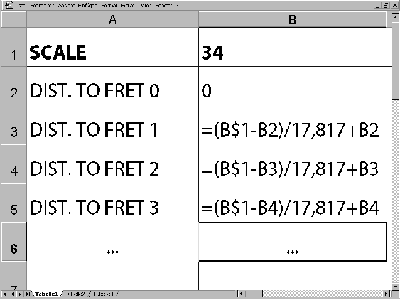Guitar building tips

# Calculating fret distances

If you divide any scale length by the constant 17.817, you will get the distance from the front edge of the nut to the first fret. Here is one example: a scale length of 25.5" (650mm) divided by 17.817 gives 1.4312173" (36.482011mm), which can be rounded down to 1.431" (36.48mm).

Three digits behind the comma are sufficient when using Imperial measurements (two when using metric units), as it is virtually impossible to place a fret much more accurately than to 1/1000 of an inch. The first fret is therefore placed at a distance of 1.431" (36.48mm) from the nut. To calculate the position of the second fret, the remaining scale length (25.5" minus 1.431" = 24.069") is again divided by 17.817. This gives the distance from the first fret at which the second fret has to be placed - in the above example this would be 1.351" (34.43mm).All fret distances should ideally be measured from the front side of the nut or a potential zero-fret, as otherwise several minor errors in the actual fretting could eventually add up to quite considerable differences.

It is consequently advisable to determine all fret positions by measuring from the nut: the first fret is placed at a distance of 1.431" from the nut, the second at 1.431" + 1.351" (=2.782") from the nut, and so on. For calculating the position of the third fret, the remaining scale length - i.e. the distance from the second fret to the bridge - is again divided by 17.817, and so on for all other frets. For the 12th fret the calculations have to give exactly half of the total scale length (at least if you ignore anything beyond the 3rd (or 2nd) digit behind the comma as there are always unavoidable rounding errors if you use a calculator or a spreadsheet program).

# How to use a spreadsheet programDoing all these calculations with a calculator can be a bit tedious, but it can easily be automated with a spreadsheet program such as Microsoft EXCEL:
Enter the scale length in field B1 assign the value "0" to B2, and enter the formula "=(B\$1-B2) / 17.817 + B2" for calculating the distance between the nut and the first fret into field B3. To calculate all other fret distances simply copy the formula of field B2 into the other fields below it. Finally number all frets in column A and then print out the sheet, checking that no errors have occurred and that, for instance, the distance calculated for the 12th fret is exactly half of the total scale length (ignore unavoidable rounding errors past the second or third digit behind the comma).

## BASIC-program for calculating fret distances for any scale length

```
CLS

A = 0
X = 0

INPUT "Scale length? ",S
INPUT "# of frets ",N

PRINT "Fret distances from the nut"
PRINT
PRINT "Scale length: ";S;" mm"
PRINT

FOR B = 1 TO N
L = S - A
X = L / 17.817
A = A + X
PRINT "Distance to fret ";B;": ";A;" mm"
NEXT B

END
```

## C-program for calculating fret distances for any scale length

Written by Chris St. Pierre
```

main()
{
float distance, scale, location, scaling_factor;
int num_frets, fret;

scaling_factor = 0;
distance = 0;

printf ("Enter the scale desired: ");
scanf ("%f", &scale);
printf ("\nEnter the number of frets: ");
scanf ("%d", &num_frets);

printf("\nFret Distances From the Nut in mm\n");
printf("=================================\n");

for (fret = 1; fret <= num_frets; fret++)
{
location = scale - distance;
scaling_factor = location / 17.817;
distance = distance + scaling_factor;
printf("Distance to fret %d in mm : %f\n", fret, distance);
}

printf("=================================\n");
return;
}

```